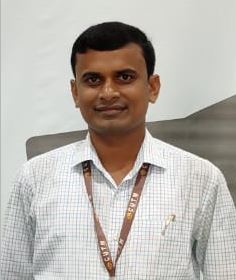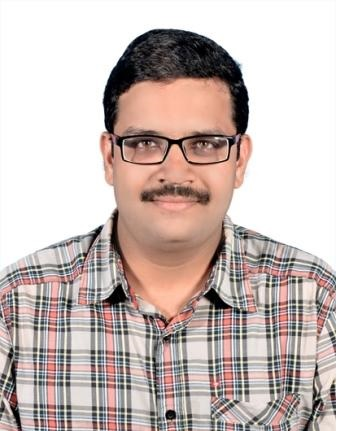Analog Communication SystemsTeacher

Dr. Harish Chandra MohantaCategory

Course Attendees

Still no participant

Still no reviews

Code(Credit) : CUTM1045 (2-1-0)

Course Objectives

• Impart the basic concepts of analog modulation schemes.
• Describe different types of noise and predict its effect on various analog communication systems.
• Know the techniques of analog communication and noise analysis in analog communication.

Learning Outcomes

Upon successful completion of this course, students should be able to:

• Analyze energy and power spectral density of the signal.
• Develop an understanding of the performance of analog communication systems.
• Calculate bandwidth and power requirements for analog systems.
• Analyze the different characteristics of the receiver.

Course Syllabus

Module I (5 Hours)

Basic block diagram of analog communication. Need for modulation, Fourier transforms, Properties of Fourier transform (Duality property, Frequency shifting property, Modulation property). Introduction to AM: Time-Domain description, Frequency – Domain description,

Generation of AM wave: square law modulator, switching modulator. Detection of AM waves: square law detector, envelop detector.

Practice: (using Hardware and MATLAB)

DSB+C Modulation using Trainer Kit and MATLAB

DSB+C Demodulation using Trainer Kit and MATLAB

Module II (6 Hours)

Double sideband suppressed carrier modulation (DSBSC): Time-Domain description, Frequency-Domain representation, Generation of DSBSC waves: balanced modulator, ring modulator. Coherent detection of DSBSC modulated waves.

SINGLE SIDE-BAND MODULATION (SSB):

Quadrature carrier multiplexing, SSB modulation, Frequency-Domain description of SSB wave, Time-Domain description. Phase discrimination method for generating an SSB modulated wave, Demodulation of SSB waves.

Practice: (using Hardware and MATLAB)

Quadrature Carrier Multiplexing using Trainer Kit and  MATLAB.

DSB-SC Modulation and Demodulation using Trainer Kit and MATLAB.

VESTIGIAL SIDE-BAND MODULATION (VSB):

Frequency – Domain description, Generation of VSB modulated wave, Time – Domain description, Frequency division multiplexing, Superheterodyne receiver.

Practice: (using Hardware and MATLAB)

Frequency Division Multiplexing using Trainer Kit and MATLAB.

Module III (5 Hours)

ANGLE MODULATION (FM):

Basic definitions, FM, narrowband FM, wideband FM, the transmission bandwidth of FM waves, Generation of FM waves: indirect FM, and direct FM.

Practice: (using Hardware and MATLAB)

FM Modulation using Trainer Kit and MATLAB

Module IV (4 Hours)

DEMODULATION OF FREQUENCY MODULATED SIGNALS:

Demodulation of FM waves, Phase-locked loop, Non-linear model of the phase-locked loop, Linear model of the phase-locked loop, Nonlinear effects in FM systems.

Practice: (using Hardware and MATLAB)

FM Demodulation using Trainer Kit and MATLAB.

FM Demodulation using PLL.

Module V (4 Hours)

RANDOM PROCESS:

Random variables, Statistical averages: Function of random variables, moments, mean, Correlation, and Covariance function: Principles of the autocorrelation function, cross-correlation functions, Central limit theorem, Properties of Gaussian process.

Practice: (Using MATLAB)

Study of Autocorrelation & Cross-correlation using MATLAB

Module VI (5 Hours)

NOISE: Introduction, shot noise, thermal noise, white noise, Noise equivalent bandwidth, Narrow bandwidth, Noise Figure,  Frequency Domain Representation of Noise, Power Spectral Density, Spectral Components of Noise, Response of a Narrowband filter to noise, Effect of a Filter on the Power spectral density of noise, Superposition of Noises, Noise Bandwidth, Narrowband representation of noise and its PSD.

Practice: (using MATLAB)

Generation of Gaussian Noise using MATLAB

Module VII (3 Hours)

NOISE IN CONTINUOUS WAVE MODULATION SYSTEMS:

Introduction, Receiver model, Noise in DSB-SC receivers, Noise in SSB receivers, Noise in AM receivers, Threshold effect, Noise in FM receivers, FM threshold effect, Pre-emphasis and De-emphasis in FM.

Reference

Text Books:

1. Communication Systems, Simon Haykins, 5th Edition, John Willey, India Pvt. Ltd, 2009.
2. Taub, D. L Schilling, G. Saha, Principles of Communication System, 3rd Edition, 2008, Tata McGraw Hill, India; ISBN: 0070648115. (Selected portions from chapters: 1, 2, 3, 4, 5, 7, 8 and 9).

Reference Books:

1. Modern Digital and Analog communication systems B. P. Lathi, Oxford University Press., 4th ed, 2010.
2. Communication System Engineering, Second Edition by MasoudSalehi, John G. Proakis, ISBN: 0130950076.
3. Principles of Electronic communication Systems, Louis E. Frenzel,3rd Edition, Tata McGraw Hill.
4. Communication Systems: Analog and digital, Singh and Sapre, TMH, 2nd Ed, 2007.

Session 1

Basic block diagram of analog communication, information source, source transducer, transmitter, channel, receiver. Need for modulation.

ACS_ Communication System Block Diagram

Session 2

Fourier Transforms properties: duality property, frequency shifting property, modulation property.

ACS_Fourier Transform and its Properties

Session 3

Introduction to AM (Time Domain & Frequency Domain Description)

ACS_ Amplitude Modulation

Session 4

Generation of AM wave: Square Law Modulator, Switching Modulator.

ACS_Generation of AM Signals

Lab Practice

AM(DSB+C) Modulation using MATLAB and Trainer Kit

http://vlab.amrita.edu/index.php?sub=59&brch=163&sim=260&cnt=2644

Session 5

Detection of AM waves: square law detector, envelop detector.

Study of DSB+C demodulation using Envelope Detector using trainer kit.

http://vlab.amrita.edu/index.php?sub=59&brch=163&sim=259&cnt=358

ACS_ Demodulation Circuit

Session 6

Double sideband suppressed carrier modulation (DSBSC): Time-Domain description, Frequency-Domain representation.

ACS_ DSBSC

Lab Practice

To Study Generation of DSB-SC signal using trainer kit and MATLAB.

Session 8

Coherent detection of DSBSC modulated waves.

ACS_ Demodulation Circuit

ACS_ COSTAS LOOP

Lab Practice

Study of coherent detection of DSB-SC  using trainer kit.

Session 9

Using MATLAB to study the Quadrature Schemes.

Session 10

Single side-band modulation, Frequency-Domain description of SSB wave, Time-Domain description.

Lab Practice

Generation of SSB using trainer kit and MATLAB.

Session 11

Phase discrimination method for generating an SSB modulated wave & Demodulation of SSB waves.

Session 12

Frequency – Domain description, Generation of VSB modulated wave, Time – Domain description, Superheterodyne Receiver

Session 13

Frequency Division Multiplexing(FDM).

Lab Practice

Simulink model design and study of FDM using MATLAB.

Session 14

Basic definitions, Frequency Modulation (FM).

ACS_ Angle Modulation (Frequency and Phase Modulation)

Session 15

Narrowband FM, Wideband FM, The transmission bandwidth of FM waves.

ACS_Frequency Modulation

Session 16

Direct Method of FM Generation.

Lab Practice

Generation of FM Signal using trainer kit and MATLAB.

Session 17

Indirect Method of FM Generation (Armstrong Method)

Session 18

Demodulation of FM waves.

ACS_ Demodulation of FM Signals

Session 19

A nonlinear model of the phase-locked loop, Linear model of the phase-locked loop, Nonlinear effects in FM systems.

Session 20

Lab Practice

FM Demodulation using Trainer Kit and MATLAB.

http://vlab.amrita.edu/index.php?sub=59&brch=163&sim=261&cnt=474

Session 21

Lab Practice

Study of FM demodulation using PLL

Session 22

Mathematical Definition of Random Process, Stationary Process.

Session 23

Random variables: Several random variables.

random Variable

Session 24

Statistical averages: Function of Random variables, moments, Mean, Mean Square value, Variance, Standard deviation.

Session 25

Correlation and Covariance function: Principles of the autocorrelation function, cross-correlation functions. Central limit theorem.

Lab Practice

Study of autocorrelation and cross-correlation using MATLAB.

Session 26

Introduction, shot noise, thermal noise, white noise, Noise equivalent bandwidth, Narrow bandwidth.

ACS_ Noise

Session 27

Noise Figure.

Lab Practice

Study of Gaussian noise using MATLAB.

Session 28

Frequency Domain Representation of Noise, Power Spectral Density, Spectral Components of Noise.

Session 29

The response of a narrowband filter to noise, the effect of a filter on the power spectral density of noise.

Session 30

Superposition of noises, Noise Bandwidth, Narrowband representation of noise, and its PSD.

Session 31

Introduction of receiver model. The figure of merit (FOM) of a receiver.

Figure merit of SSB receiver Derivation

Session 33

Noise in AM receivers, Threshold effect. Pre-emphasis and De-emphasis in FM.

Our Main TeachersDr. Harish Chandra Mohanta

Assistant Professor, ECE Department, Bhubaneswar Campus, CUTM
VIEW PROFILE

I received my M. Tech degree in 2015 from IIT, Kharagpur and PhD degree from Deakin University, Australia in 2021. Now, I am working as an Asst. Professor in ECE Department, SoET, Bhubaneswar campus, CUTM.   Research Interests: Wireless Energy Harvesting System. Metamaterial Study. Printed Microstrip Antenna Design. Transceiver System Design. Biomedical Instrumentation. AI […]Prabhat K. Patnaik

Asst. Professor in School of Engineering and Technology Department of ECE
VIEW PROFILE

Prabhat K. Patnaik received a M.Tech. from Berhampur University in 2012 and B.Tech from Biju Patnaik University of Technology and Management in 2007.Presently he is working as Asst. Professor in Electronics and Communication Engineering Department at Centurion University of Technology and Management, Odisha, India. He has published 10 research articles. His current research includes digital […]